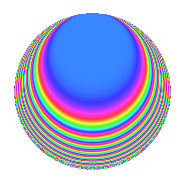# Properties

 Label 4032.2.i.aLevel 4032 Weight 2 Character orbit 4032.i Analytic conductor 32.196 Analytic rank 0 Dimension 8 CM No Inner twists 8

# Related objects

## Newspace parameters

 Level: $$N$$ = $$4032 = 2^{6} \cdot 3^{2} \cdot 7$$ Weight: $$k$$ = $$2$$ Character orbit: $$[\chi]$$ = 4032.i (of order $$2$$ and degree $$1$$)

## Newform invariants

 Self dual: No Analytic conductor: $$32.195682095$$ Analytic rank: $$0$$ Dimension: $$8$$ Coefficient field: 8.0.40960000.1 Coefficient ring: $$\Z[a_1, \ldots, a_{23}]$$ Coefficient ring index: $$2^{8}$$ Sato-Tate group: $\mathrm{SU}(2)[C_{2}]$

## $q$-expansion

Coefficients of the $$q$$-expansion are expressed in terms of a basis $$1,\beta_1,\ldots,\beta_{7}$$ for the coefficient ring described below. We also show the integral $$q$$-expansion of the trace form.

 $$f(q)$$ $$=$$ $$q$$ $$- \beta_{1} q^{5}$$ $$+ ( - \beta_{2} + \beta_{4} ) q^{7}$$ $$+O(q^{10})$$ $$q$$ $$- \beta_{1} q^{5}$$ $$+ ( - \beta_{2} + \beta_{4} ) q^{7}$$ $$+ \beta_{3} q^{11}$$ $$+ 2 \beta_{2} q^{17}$$ $$+ 2 \beta_{7} q^{19}$$ $$- \beta_{6} q^{23}$$ $$+ q^{25}$$ $$+ \beta_{7} q^{29}$$ $$-4 \beta_{4} q^{31}$$ $$+ ( 2 \beta_{3} + \beta_{5} ) q^{35}$$ $$- \beta_{5} q^{37}$$ $$+ 2 \beta_{2} q^{41}$$ $$-2 \beta_{1} q^{43}$$ $$-8 q^{47}$$ $$+ ( 3 + 2 \beta_{6} ) q^{49}$$ $$+ 3 \beta_{7} q^{53}$$ $$-2 \beta_{4} q^{55}$$ $$-2 \beta_{5} q^{59}$$ $$+ 6 \beta_{3} q^{61}$$ $$+ \beta_{1} q^{67}$$ $$-3 \beta_{6} q^{71}$$ $$-2 \beta_{6} q^{73}$$ $$+ ( \beta_{1} + \beta_{7} ) q^{77}$$ $$-4 \beta_{2} q^{79}$$ $$-2 \beta_{5} q^{85}$$ $$-6 \beta_{2} q^{89}$$ $$-4 \beta_{6} q^{95}$$ $$+ 2 \beta_{6} q^{97}$$ $$+O(q^{100})$$ $$\operatorname{Tr}(f)(q)$$ $$=$$ $$8q$$ $$\mathstrut +\mathstrut O(q^{10})$$ $$8q$$ $$\mathstrut +\mathstrut 8q^{25}$$ $$\mathstrut -\mathstrut 64q^{47}$$ $$\mathstrut +\mathstrut 24q^{49}$$ $$\mathstrut +\mathstrut O(q^{100})$$

Basis of coefficient ring in terms of a root $$\nu$$ of $$x^{8}\mathstrut +\mathstrut$$ $$7$$ $$x^{4}\mathstrut +\mathstrut$$ $$1$$:

 $$\beta_{0}$$ $$=$$ $$1$$ $$\beta_{1}$$ $$=$$ $$($$$$2 \nu^{6} + 16 \nu^{2}$$$$)/3$$ $$\beta_{2}$$ $$=$$ $$($$$$2 \nu^{4} + 7$$$$)/3$$ $$\beta_{3}$$ $$=$$ $$($$$$2 \nu^{7} - \nu^{5} + 13 \nu^{3} - 5 \nu$$$$)/3$$ $$\beta_{4}$$ $$=$$ $$($$$$-2 \nu^{7} - \nu^{5} - 13 \nu^{3} - 5 \nu$$$$)/3$$ $$\beta_{5}$$ $$=$$ $$2 \nu^{6} + 12 \nu^{2}$$ $$\beta_{6}$$ $$=$$ $$($$$$4 \nu^{7} + \nu^{5} + 29 \nu^{3} + 11 \nu$$$$)/3$$ $$\beta_{7}$$ $$=$$ $$($$$$-4 \nu^{7} + \nu^{5} - 29 \nu^{3} + 11 \nu$$$$)/3$$
 $$1$$ $$=$$ $$\beta_0$$ $$\nu$$ $$=$$ $$($$$$\beta_{7}\mathstrut +\mathstrut$$ $$\beta_{6}\mathstrut +\mathstrut$$ $$\beta_{4}\mathstrut +\mathstrut$$ $$\beta_{3}$$$$)/4$$ $$\nu^{2}$$ $$=$$ $$($$$$-$$$$\beta_{5}\mathstrut +\mathstrut$$ $$3$$ $$\beta_{1}$$$$)/4$$ $$\nu^{3}$$ $$=$$ $$($$$$-$$$$\beta_{7}\mathstrut +\mathstrut$$ $$\beta_{6}\mathstrut +\mathstrut$$ $$2$$ $$\beta_{4}\mathstrut -\mathstrut$$ $$2$$ $$\beta_{3}$$$$)/2$$ $$\nu^{4}$$ $$=$$ $$($$$$3$$ $$\beta_{2}\mathstrut -\mathstrut$$ $$7$$$$)/2$$ $$\nu^{5}$$ $$=$$ $$($$$$-$$$$5$$ $$\beta_{7}\mathstrut -\mathstrut$$ $$5$$ $$\beta_{6}\mathstrut -\mathstrut$$ $$11$$ $$\beta_{4}\mathstrut -\mathstrut$$ $$11$$ $$\beta_{3}$$$$)/4$$ $$\nu^{6}$$ $$=$$ $$($$$$4$$ $$\beta_{5}\mathstrut -\mathstrut$$ $$9$$ $$\beta_{1}$$$$)/2$$ $$\nu^{7}$$ $$=$$ $$($$$$13$$ $$\beta_{7}\mathstrut -\mathstrut$$ $$13$$ $$\beta_{6}\mathstrut -\mathstrut$$ $$29$$ $$\beta_{4}\mathstrut +\mathstrut$$ $$29$$ $$\beta_{3}$$$$)/4$$

## Character Values

We give the values of $$\chi$$ on generators for $$\left(\mathbb{Z}/4032\mathbb{Z}\right)^\times$$.

 $$n$$ $$127$$ $$577$$ $$1793$$ $$3781$$ $$\chi(n)$$ $$1$$ $$-1$$ $$-1$$ $$-1$$

## Embeddings

For each embedding $$\iota_m$$ of the coefficient field, the values $$\iota_m(a_n)$$ are shown below.

For more information on an embedded modular form you can click on its label.

Label $$\iota_m(\nu)$$ $$a_{2}$$ $$a_{3}$$ $$a_{4}$$ $$a_{5}$$ $$a_{6}$$ $$a_{7}$$ $$a_{8}$$ $$a_{9}$$ $$a_{10}$$
1889.1
 0.437016 + 0.437016i −0.437016 − 0.437016i −1.14412 − 1.14412i 1.14412 + 1.14412i −0.437016 + 0.437016i 0.437016 − 0.437016i 1.14412 − 1.14412i −1.14412 + 1.14412i
0 0 0 2.00000i 0 −2.23607 1.41421i 0 0 0
1889.2 0 0 0 2.00000i 0 −2.23607 + 1.41421i 0 0 0
1889.3 0 0 0 2.00000i 0 2.23607 1.41421i 0 0 0
1889.4 0 0 0 2.00000i 0 2.23607 + 1.41421i 0 0 0
1889.5 0 0 0 2.00000i 0 −2.23607 1.41421i 0 0 0
1889.6 0 0 0 2.00000i 0 −2.23607 + 1.41421i 0 0 0
1889.7 0 0 0 2.00000i 0 2.23607 1.41421i 0 0 0
1889.8 0 0 0 2.00000i 0 2.23607 + 1.41421i 0 0 0
 $$n$$: e.g. 2-40 or 990-1000 Embeddings: e.g. 1-3 or 1889.8 Significant digits: Format: Complex embeddings Normalized embeddings Satake parameters Satake angles

## Inner twists

Char. orbit Parity Mult. Self Twist Proved
1.a Even 1 trivial yes
8.b Even 1 yes
12.b Even 1 yes
21.c Even 1 no
24.f Even 1 yes
28.d Even 1 no
56.e Even 1 no
168.i Even 1 no

## Hecke kernels

This newform can be constructed as the intersection of the kernels of the following linear operators acting on $$S_{2}^{\mathrm{new}}(4032, \chi)$$:

 $$T_{5}^{2}$$ $$\mathstrut +\mathstrut 4$$ $$T_{47}$$ $$\mathstrut +\mathstrut 8$$